Circles - Olympiad Level MCQ, Class 10 Mathematics

# Circles - Olympiad Level MCQ, Class 10 Mathematics

Test Description

## 25 Questions MCQ Test Olympiad Preparation for Class 10 | Circles - Olympiad Level MCQ, Class 10 Mathematics

Circles - Olympiad Level MCQ, Class 10 Mathematics for Class 10 2022 is part of Olympiad Preparation for Class 10 preparation. The Circles - Olympiad Level MCQ, Class 10 Mathematics questions and answers have been prepared according to the Class 10 exam syllabus.The Circles - Olympiad Level MCQ, Class 10 Mathematics MCQs are made for Class 10 2022 Exam. Find important definitions, questions, notes, meanings, examples, exercises, MCQs and online tests for Circles - Olympiad Level MCQ, Class 10 Mathematics below.
 1 Crore+ students have signed up on EduRev. Have you?
Circles - Olympiad Level MCQ, Class 10 Mathematics - Question 1

### ABCD is a cyclic quadrilateral PQ is a tangent at B. If ∠DBQ = 65° , then ∠BCD is :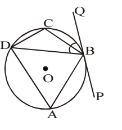Circles - Olympiad Level MCQ, Class 10 Mathematics - Question 2

### In the given figure, AP = 2 cm, BP = 6 cm and CP = 3 cm. Find DP :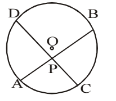Circles - Olympiad Level MCQ, Class 10 Mathematics - Question 3

### In the given figure, AP = 3 cm, BA = 5 cm and CP = 2 cm. Find CD :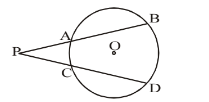Circles - Olympiad Level MCQ, Class 10 Mathematics - Question 4

In the given figure, tangent PT = 5 cm, PA = 4 cm, find AB :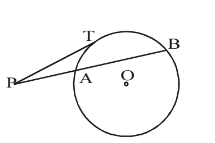Circles - Olympiad Level MCQ, Class 10 Mathematics - Question 5

Two circles of radii 13 cm and 5 cm touch internally each other. Find the distance between their centres :

Circles - Olympiad Level MCQ, Class 10 Mathematics - Question 6

Three circles touch each other externally. The distance between their centre is 5 cm, 6 cm and 7 cm. Find the radii of the circles :

Circles - Olympiad Level MCQ, Class 10 Mathematics - Question 7

If AB is a chord of a circle, P and Q are two points on the circle different from A and B, then:

Circles - Olympiad Level MCQ, Class 10 Mathematics - Question 8

In the given figure, CD is a direct common tangent to two circles intersecting each other at A and B, then: ∠CAD + ∠CBD = ?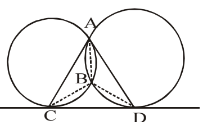Circles - Olympiad Level MCQ, Class 10 Mathematics - Question 9

In a circle of radius 5 cm, AB and AC are the two chords such that AB = AC = 6 cm. Find the length of the chord BC.

Circles - Olympiad Level MCQ, Class 10 Mathematics - Question 10

In a circle of radius 17 cm, two parallel chords are drawn on opposite sides of a diameter. The distance between the chords is 23 cm. If the length of one chord is 16 cm, then the length of the other is :

Circles - Olympiad Level MCQ, Class 10 Mathematics - Question 11

If two circles are such that the centre of one lies on the circumference of the other, then the ratio of the common chord of two circles to the radius of any of the circles is :

Circles - Olympiad Level MCQ, Class 10 Mathematics - Question 12

Two circles touch each other internally. Their radii are 2 cm and 3 cm. The biggest chord of the other circle which is outside the inner circle, is of length :

Circles - Olympiad Level MCQ, Class 10 Mathematics - Question 13

Through any given set of four points P,Q, R, S it is possible to draw :

Circles - Olympiad Level MCQ, Class 10 Mathematics - Question 14

What is the probability, that a number selected from 1, 2, 3, …, 25, is a prime number, when each of the numbers is equally likely to be selected.

Detailed Solution for Circles - Olympiad Level MCQ, Class 10 Mathematics - Question 14

S = { 1, 2, 3, ---- , 25} |S|= 25 And E = { 2, 3, 5, 7, 11, 13, 17, 19, 23 } |E|= 9 Hence P(E) = |E| / |S| = 9 / 25

Circles - Olympiad Level MCQ, Class 10 Mathematics - Question 15

The number of common tangents that can be drawn to two given circles is at the most :

Detailed Solution for Circles - Olympiad Level MCQ, Class 10 Mathematics - Question 15

When two circles touch each other externally, 3 common tangents can be drawn to the circles.

When two circle neither touch nor intersect and one lies outside the other, then 4 common tangents can be drawn.

Circles - Olympiad Level MCQ, Class 10 Mathematics - Question 16

ABC is a right angled triangle AB = 3 cm, BC = 5 cm and AC = 4 cm, then the inradius of the circle is :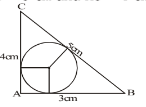Circles - Olympiad Level MCQ, Class 10 Mathematics - Question 17

In the given figure O is the centre of the circle and ∠BAC = 25°, then the value of ∠ADB is :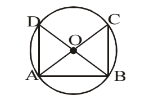Circles - Olympiad Level MCQ, Class 10 Mathematics - Question 18

In the given circle O is the centre of the circle and AD, AE are the two tangents. BC is also a tangent, then :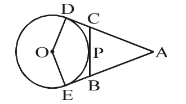Circles - Olympiad Level MCQ, Class 10 Mathematics - Question 19

In a circle O is the centre and ∠COD is right angle. AC = BD and CD is the tangent at P. What is the value of AC + CP, if the radius of the circle is 1 metre?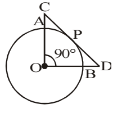Circles - Olympiad Level MCQ, Class 10 Mathematics - Question 20

In a triangle ABC, O is the centre of incircle PQR, ∠BAC = 65°, ∠BCA = 75°, find ∠ROQ :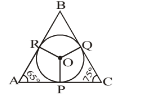Circles - Olympiad Level MCQ, Class 10 Mathematics - Question 21

In the adjoining figure O is the centre of the circle. ∠AOD = 120°. If the radius of the circle be 'r', then find the sum of the areas of quadrilaterals AODP and OBQC :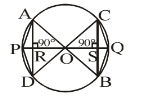Circles - Olympiad Level MCQ, Class 10 Mathematics - Question 22

There are two circles each with radius 5 cm. Tangent AB is 26 cm. The length of tangent CD is :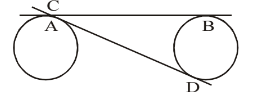Circles - Olympiad Level MCQ, Class 10 Mathematics - Question 23

In the adjoining figure O is the centre of the circle and AB is the diameter. Tangent PQ touches the circle at D. ∠BDQ = 48°. Find the ratio of ∠DBA : ∠DCB :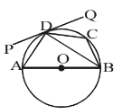Circles - Olympiad Level MCQ, Class 10 Mathematics - Question 24

In the given diagram an incircle DEF is circumscribed by the right angled triangle in which AF = 6 cm and EC = 15 cm. Find the difference between CD and BD :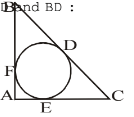Circles - Olympiad Level MCQ, Class 10 Mathematics - Question 25

In the adjoining figure 'O' is the centre of circle, ∠CAO = 25° and ∠CBO = 35°. What is the value of ∠AOB?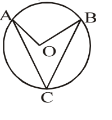## Olympiad Preparation for Class 10

39 tests
 Use Code STAYHOME200 and get INR 200 additional OFF Use Coupon Code